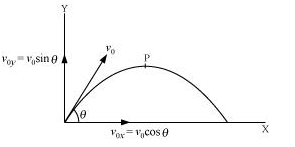## Pages

### Motion in a plane NCERT Solutions Class 11 Physics - Solved Exercise Question 4.32

Question 4.32:

(a) Show that for a projectile the angle between the velocity and the x-axis as a function of time is given by
θ(t) = tan-1((v0y- gt) / v0x)
(b) Shows that the projection angle θ0 for a projectile launched from the origin is given by
θ0 = tan-1(4hm / R)
where the symbols have their usual meaning.

Solution:

(a) Let v0x and v0y respectively be the initial components of the velocity of the projectile along horizontal (x) and vertical (y) directions.
Let vx and vy respectively be the horizontal and vertical components of velocity at a point P.Time taken by the projectile to reach point P = t
Applying the first equation of motion along the vertical and horizontal directions, we get:
vy = v0y = gt
And vx = v0x
∴ tan θ = vy / vx = (v0y - gt) / v0x
θ = tan-1 (v0y - gt) / v0x
(b) Maximum vertical height, hm = u02 Sin2θ / 2g   ...(i)
Horizontal range, R = u02 Sin22θ / g   ... (ii)
hm / R = Sin2θ / 2Sin22θ
= Sin θ X Sin θ / 2 X 2SinθCosθ
= Sin θ / 4 Cos θ = tan θ / 4
tan θ = (4hm / R)
θ = tan-1 (4hm / R)